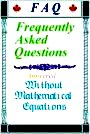Relationship between E=mc2 and m=gmo

( Last checked 2017/01/15 - The estate of Paul Marmet )
Go to: Next series of questions

Previous series of questions
Series #13A -                    Origin of E=Mc2
-----------------------
E=Mc2 before Albert Einstein.

When you read the original papers on relativity and physics, we find that E=Mc2 is a relationship, which is much older than Einstein. This relationship was not included within the original Einstein paper but in a later paper.  The relation between mass and energy began in 1881 with Thomson’s electromagnetic mass and was modified in Heaviside formula in 1889.  Poincarré in 1900 represented the mass by r and the energy density J of a fictitious fluid of radiation by the equation J=r/c2.  In 1904 Hasenorhl showed that the energy in a moving cavity would increase by 8E/(3c3), which Abraham persuaded him to change for 4E/(2c3). Also Soddy in 1904 suggested that the process of radioactive decay involves a conversion of mass into energy.  But the idea of E=mc2 as a potential energy for the potential of light is also explicit in Newtonian corpuscular theory.  In Newton`s Query 30, we read: "Are not gross Bodies and Light convertible into one another, . . . ".
Books relating historical facts give the correct facts about science.  About the History of Science, I suggest that you should read the Book "A Revolution Too Far" (1994) by Dr. Peter Rowland  (PD Publications, 2 Ascot Park, Liverpool, L23 2XH).
My book is based on the conservation of mass-energy. This idea is required by the philosophical principle of Causality. I believe that nothing can happen without a “Cause”.  In nature, we can just transform mass into energy and energy into mass. We cannot create energy or mass from nothing. Of course, we need a constant of proportionality between mass and energy.  The constant of proportionality is c2.  Surprisingly, Einstein`s general relativity is not compatible with the principle of mass-energy conservation (see reference).  That is a fatal error.  That is illogical. Furthermore, special relativity is not much useful, because it cannot deal with gravity or the acceleration of matter.

The fact that the relationship E=mc2 was not derived by Einstein is also discussed by H. E. Yves in “Derivation of the Mass-Energy Relationship” Journal of the Optical Society of America” Vol. 42. No: 8, August 1952. pp. 540-543.  Also a similar discussion is presented by Christopher Jon Bjerknes in the Book: “Albert Einstein the Incorrigible Plagiarist” Ed. XTX INC, P.O. Box 9361, Downers Grove, Illinois 60515, U.S.A.  Chapter 5, pp161-168 (2002).

==========================

Question - 13B
From E=Mc2 to m= g mo
Using the principle of "mass-energy conservation" represented by equation E=Mc2, we can calculate the increase of mass given to a particle due to its increase of kinetic energy?  That demonstration is added here to complete chapters 2 and 3 of the book Einstein’s Theory of Relativity Versus Classical Mechanics.  Dr. Robert E. LeChevalier noted that this calculation would be appropriate in these chapters.  Since this relationship is not indicated in chapters 2 and 3, let us present this demonstration here.
The conventional derivation of that relationship is the following.
We have:1
also2
Equations 1 and 2 give: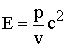3
Also, we have: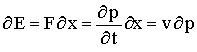4
Equations 1 and 4 give:5
Let us integrate equation 5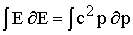6
Equation 6 gives:7
Where,, is the integrating constant, corresponding to the rest energy.  Equation 3 also gives: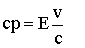8
Equation 8 in 7 gives: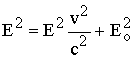9
Equation 9 gives10
Substituting the mass-energy relationship Eo=moc2 and E=mc2, equations 1 and 10 give:11
Equation 11 shows that the increase of mass of a moving particle is a direct consequence of mass-energy conservation. This is the demonstration of the relationship used in chapter 2 and 3 of the book: “Einstein’s Theory of Relativity Versus Classical Mechanics”.

<><><><><><><><><><><><>

New Choice of Questions
<><><><><><><><><><><><><>